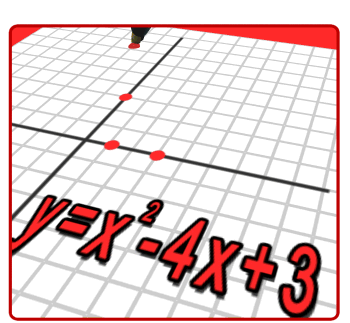COMMITMENT HONOR SUCCESSScience • Technology • Engineering • Art • Math
•
Andrew Lapic
Mathematics TeacherAPEX
Period 0

Algebra 1
Period 1 & 2
Algebra 1 introduces students to variables, algebraic expressions, equations, inequalities, functions, and all their multiple representations. In this class, students will develop the ability to explore and solve real-world application problems, demonstrate the appropriate use of calculators, and communicate mathematical ideas clearly. This course lays the foundation for mathematical literacy that will help students be successful in every subsequent course in mathematics.

Introduction to Data Science
Period 3 & 4
Introduction to Data Science (IDS) is designed to introduce students to the exciting opportunities available at the intersection of data analysis, computing, and mathematics through hands-on activities. Data is everywhere, and this curriculum will help prepare students to live in a world of data. The curriculum focuses on practical applications of data analysis to give students concrete and applicable skills. Instead of using small, tailored, curated data sets as in a traditional statistics curriculum, this curriculum engages students with a wider world of data that fall into the "Big Data" paradigm and are relevant to students' lives. In contrast to the traditional formula-based approach, in IDS, statistical inference is taught algorithmically, using modern randomization and simulation techniques. Students will learn to find and communicate meaning in data, and to think critically about arguments based on data.